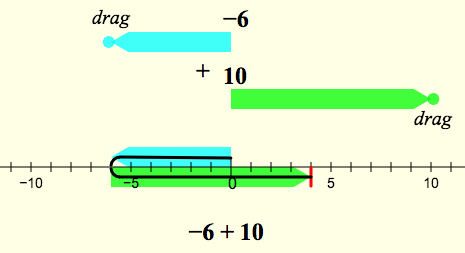A recent post on the my NCTM discussion group asked about tools to help students visualize and understand addition and subtraction of integers.

I always found this confusing for some of my Algebra 1 students, mainly because they had been told to memorize some rules about whether to add or subtract the two integers and how to decide what sign to put on the answer. So we created animated Sketchpad models that show the two numbers moving, one after the other, to the number line. The screen captures below show how each model appears after its animation finishes:Below (and here) is the live model, converted to Web Sketchpad.

We wanted to make this visualization work as a tool for students to use in multiple ways.
Drag the two circles to represent a problem and then press the numbered buttons.
Reset to start a new problem.
Use the Present All button to show the animated steps one after the other.
Without resetting, drag the circles to observe how the model changes consistently as you change the problem.

An important objective of this pair of models is to encourage students to develop a single consistent number-line visualization for both addition and subtraction, and to realize that there’s only one difference between the two models: to subtract you just negate (flip) the second number and then add it.

Importantly, the animation never actually displays the answer; this visualization is designed to emphasize the process, and the student must determine the answer herself by inspecting the model.

When using this or any similar model, it can be very useful to require students to draw the model first, before writing down the answer to the problem.The physical act of drawing is an aid in helping students to understand and remember the connection between the model and the answer. By omitting the ticks and labels on the number line, the drawing helps students strip non-essential elements of the model and develop a robust abstract understanding.

You’ll know your students have “gotten it” when they can look at a problem, “see” the two operands appear on an imaginary number line, and immediately write down the sum or difference.